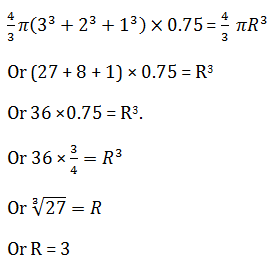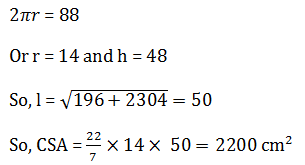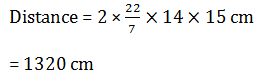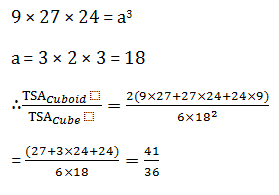# SSC Quantitative Aptitude Questions (Day-66)

Dear Aspirants, you can find the Quantitative Aptitude questions with detailed explanations for the SSC exams. Nowadays the competitive level of the exam has been increasing consistently. Due to the great demand for the government job, the level of the toughness reached greater. Candidates have to enhance the preparation process in order to drive in the right path. It doesn’t need to clear the prescribed cutoff. You must have to score good marks more than the cut off marks to get into the final provisional list. Here we have updating the Quantitative Aptitude questions with detailed explanations on a daily basis. You can practice with us and measure your level of preparation. According to that you can sculpt yourself in a proper way. SSC aspirants kindly make use of it and grab your success in your career.

Start Quiz

1) A certain sum becomes Rs 840 in 3 years and Rs 1200 in 7 years at simple interest. What is the value (in Rs.) of principal?

(a) 520

(b) 570

(c) 620

(d) 670

Direction (2-5): Thebar graph given below represents the number of players of a college taking part in three games for 3 years.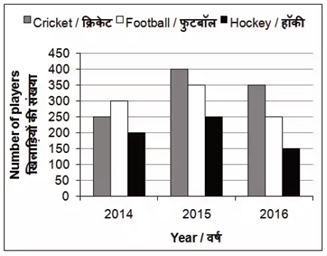2) What is the total number of players playing all three games in 2015?

(a) 950

(b) 900

(c) 1000

(d) 1050

3) Number of players playing football in 2016 is how much percent less than the players playing football in 2014?

(a) 14.28

(b) 16.66

(c) 33.33

(d) 8.33

4) Number of players playing hockey in 2015 is what percent of total players playing all the three games in 2015?

(a) 25

(b) 33.33

(c) 22

(d) 28

5) Total number of players in 2015 is what percent of total number of players in 2016?

(a) 75

(b) 112.5

(c) 141.11

(d) 133.33

6) If diagonals of a rhombus are 36 cm and 48 cm, then what is the perimeter (in cm) of the rhombus?

(a) 30

(b) 60

(c) 120

(d) 240

7) Three spherical balls of radius 3 cm, 2 cm and 1 cm are melted to form a new spherical ball. In this process there is a loss of 25% of the material. What isthe radius (in cm) of the new ball?

(a) 5

(b) 3

(c) 6

(d) 8

8) The perimeter of base of a right circular cone is 88 cm. If the height of the cone is 48 cm, then what is the curved surface area (in cm(b) of the cone?

(a) 550

(b) 1100

(c) 2200

(d) 2700

9) The radius of a wheel is 14 cm. What is the distance (in cm) travelled by the wheel in 15 revolutions?

(a) 440

(b) 660

(c) 1320

(d) 1980

10) A cuboid of sides 9 cm, 27 cm and 24 cm is melted to form a cube. What is the ratio between the total surface area of the cuboid and that of the cube?

(a) 41 : 35

(b) 41 : 36

(c) 47 : 38

(d) 49 : 36

S.I for 4 years = 1200 –  840 = 360

S.I for 3 years=270

Principal = 840 – 270 =570

400 + 350 + 250 = 1000

F2014 = 300

So, (F2014  – F2016) / F2014 =50/300 × 100 = 16.66%

H2015 = 250

All Sports 2015 = 1000

∴ % = (250 × 100)/1000 = 25%

All Sports (2015) = 1000

d All sports (2016) = 750

∴ 1000/750 × 100

400/3 = 133.33%

Clearly,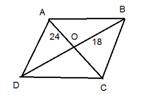AO = 24 and OB = 18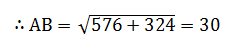So, Perimeter = 120